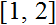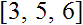# Volume 13, 2007, Number 3

Volume 13Number 1Number 2 ▷ Number 3 ▷ Number 4

Arithmetical function characterizations and identities induced through equivalence relations
Original research paper. Pages 1—19
Temba Shonhiwa
Full paper (6018 Kb) | Abstract

Let A denote the set of arithmetic functions and ∗ Dirichlet convolution. The paper presents and alternative approach to the study of arithmetic functions by introducing a homomorphism between the subgroup <U, ∗> of the group of units in <A, ∗> and the quotient ring induces through an equivalence relation. The same notion is extended to the case of unitary convolution.

On some Pascal’s like triangles. Part 3
Original research paper. Pages 20—26
Krassimir T. Atanassov
Full paper (87 Kb) | Abstract

In a series of papers, starting with, we discuss new types of Pascal’s like triangles. Triangles of the present form, but not with the present sense, are described in different publications, e.g., but at least the author had not found a research with similar idea.

In the first part of our research we studied properties of some standard sequences and in the second part — of some “special” sequences. Now, we shall construct (0, 1)-analogous of the Pascal’s like triangles (or “(mod 2)-triangles”) from the both previous papers, i.e., we will construct (mod 2)-values of their elements and will discuss the obtained configurations. We will call the new triangles “(0, 1)-triangles”.

Modular-ring class structures of xn ± yn
Original research paper. Pages 27—35
J. V. Leyendekkers and A. G. Shannon
Full paper (355 Kb) | Abstract

Integer structure theory is used to analyse the factors of sums and differences of two identical powers of two integers, x and y. For instance, the sum of two identical powers, m, cannot form primes when m is odd or when m is even if the powers are odd and of the form m/2. The expanded forms of the factors indicate why the structure acts against the sum ever equalling an identical power. The difference of odd powers can yield primes when xy = 1. The difference of even powers cannot yield primes whereas the sum can when m/2n is even. However, x2y2 can equal a prime when xy = 1.

Remark on Jacobsthal numbers
Original research paper. Page 36
Krassimir T. Atanassov
Full paper (83 Kb)

Volume 13Number 1Number 2 ▷ Number 3 ▷ Number 4